# 1470 Can You Find Factor Pairs That Make Sum-Difference?

### Today’s Puzzles:

I bet you can find a factor pair of 30 that adds up to 13 as well as another factor pair of 30 that subtracts to give you 13.

If you can solve that simple puzzle, then you will be able to solve the puzzle next to it. Even though 1470 has 12 different factor pairs, you don’t have to worry too much about them: All of the answers to the second puzzle are just _____ times the answers to the first puzzle! (And 1470 is _____² times 30.)Likewise, don’t get scared off with this next set of puzzles one of which wants you to find the factor pairs of 518616 that add up or subtract to 1470. Crazy, right? Again, if you can solve the first puzzle in the set, and if you can multiply a 3-digit number by a 1-digit number, you can easily solve the second puzzle because the answers are just _________ times the answers to the first puzzle in the set! (And 518616 is merely _________² times 6.)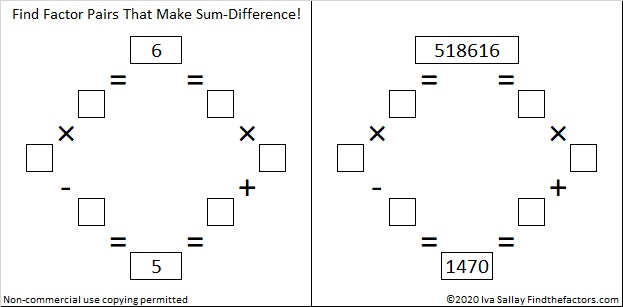If you need more help than what I’ve already said, scroll down to the factor trees for 1470. I selected those particular trees for a reason!

### Factors of 1470:

• 1470 is a composite number.
• Prime factorization: 1470 = 2 × 3 × 5 × 7 × 7, which can be written 1470 = 2 × 3 × 5 × 7²
• 1470 has at least one exponent greater than 1 in its prime factorization so √1470 can be simplified. Taking the factor pair from the factor pair table below with the largest square number factor, we get √1470 = (√49)(√30) = 7√30
• The exponents in the prime factorization are 1, 1, 1, and 2. Adding one to each exponent and multiplying we get (1 + 1)(1 + 1)(1 + 1)(2 + 1) = 2 × 2 × 2 × 3 = 24. Therefore 1470 has exactly 24 factors.
• The factors of 1470 are outlined with their factor pair partners in the graphic below.### A Few Factor Trees for 1470:### More Facts about the Number 1470:

1470 is the average of 14² and 14³. That simple fact makes 1470 the 14th Pentagonal Pyramidal Number.

1470 is the hypotenuse of a Pythagorean triple:
882-1176-1470 which is (3-4-5) times 294

Hmm… that same factor pair showed up again!

# 1469 and Level 2

### Today’s Puzzle:

Where do the Factors 1-12 belong on this multiplication table puzzle?### Factors of 1469:

• 1469 is a composite number.
• Prime factorization: 1469 = 13 × 113
• 1469 has no exponents greater than 1 in its prime factorization, so √1469 cannot be simplified.
• The exponents in the prime factorization are 1, and 1. Adding one to each exponent and multiplying we get (1 + 1)(1 + 1) = 2 × 2 = 4. Therefore 1469 has exactly 4 factors.
• The factors of 1469 are outlined with their factor pair partners in the graphic below.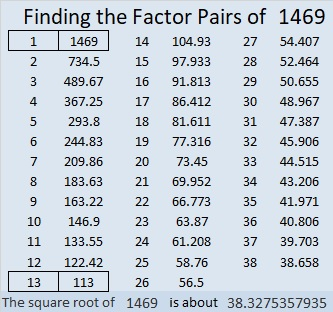### Other Facts about the Number 1469:

1469 is the sum of two squares in two different ways:
38² + 5² = 1469
37² + 10² = 1469

1469 is the hypotenuse of FOUR Pythagorean triples:
195-1456-1469 which is 13 times (15-112-113)
380-1419-1469 calculated from 2(38)(5), 38² – 5², 38² + 5²
565-1356-1469 which is (5-12-13) times 113
740-1269-1469 calculated from 2(37)(10), 37² – 10², 37² + 10²

# 1468 and Level 1

### Today’s Puzzle

Can you find the factors from 1 to 12 that will work with the given clues to turn this puzzle into a multiplication table?### Factors of 1468

• 1468 is a composite number.
• Prime factorization: 1468 = 2 × 2 × 367, which can be written 1468 = 2² × 367
• 1468 has at least one exponent greater than 1 in its prime factorization so √1468 can be simplified. Taking the factor pair from the factor pair table below with the largest square number factor, we get √1468 = (√4)(√367) = 2√367
• The exponents in the prime factorization are 2 and 1. Adding one to each exponent and multiplying we get (2 + 1)(1 + 1) = 3 × 2 = 6. Therefore 1468 has exactly 6 factors.
• The factors of 1468 are outlined with their factor pair partners in the graphic below.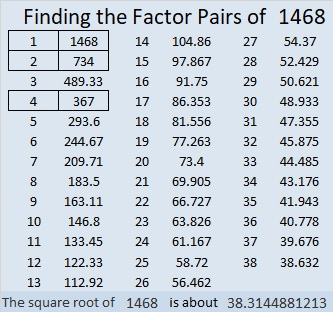### Another Interesting Fact about the number 1468:

Stetson.edu informs us that the smallest 20-digit 6th power is 1468⁶.

# 1466 A Non-Simplified Yet Easy-to-Remember Unit Circle

### Look at the Patterns in this Unit Circle:

A unit circle has a radius of one and is often used to display the sines and cosines of special angles. I’ve made a unit circle that showcases the patterns that make memorizing how to complete one easier.

This unit circle is easy to memorize because many of the values have not been simplified. However, always remember to simplify the values when giving your final answer on any assignment or test!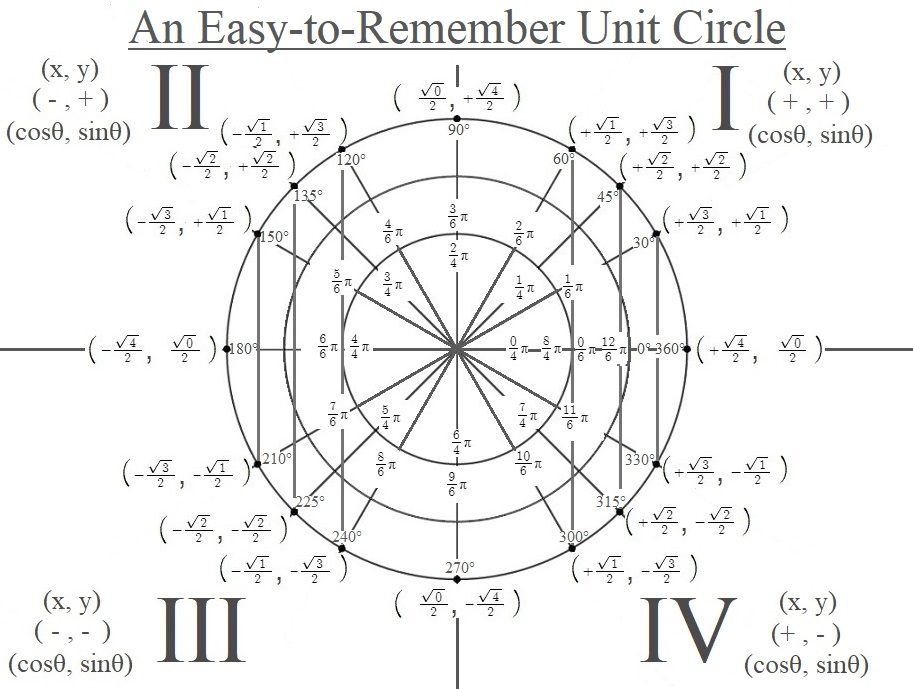The patterns you see in the ordered pairs representing the cosines and sines are only coincidental: they are merely an aid somebody else created to make memorizing the values easier. Over half of the square roots can be simplified and should be. However, I do love them in their non-simplified form because it is easy to remember them, and it is easy to see the sine wave and the cosine wave as the square root values increase and decrease incrementally as we go around the unit circle.

I’ve also made a similar unit circle that might help students locate the angles in the quadrants better: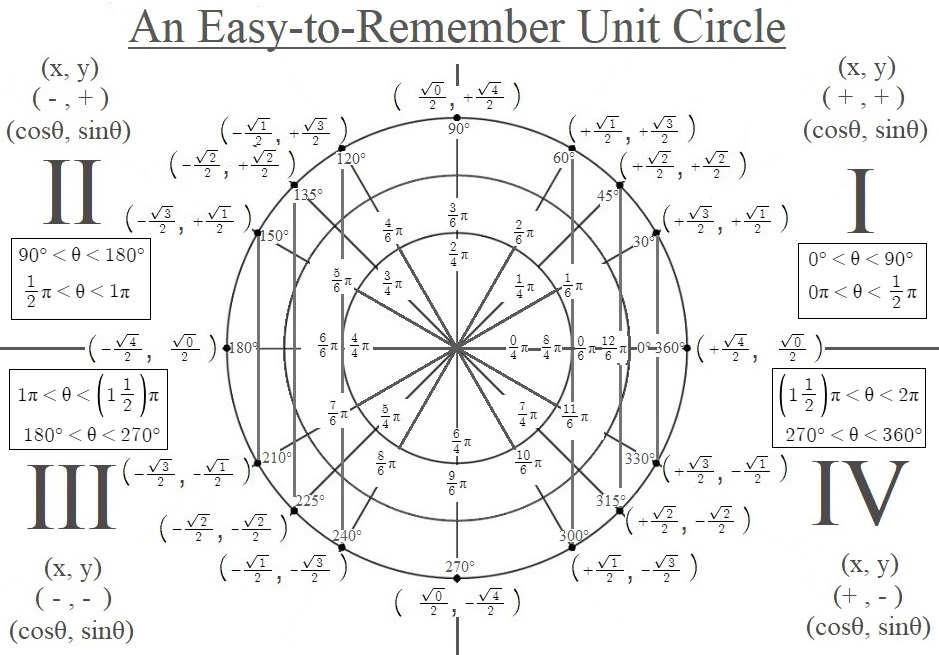### Factors of 1466:

• 1466 is a composite number.
• Prime factorization: 1466 = 2 × 733
• 1466 has no exponents greater than 1 in its prime factorization, so √1466 cannot be simplified.
• The exponents in the prime factorization are 1, and 1. Adding one to each exponent and multiplying we get (1 + 1)(1 + 1) = 2 × 2 = 4. Therefore 1466 has exactly 4 factors.
• The factors of 1466 are outlined with their factor pair partners in the graphic below.### More Facts about the number 1466:

1466 is the sum of two squares:
29² + 25² = 1466

1466 is also the hypotenuse of a Pythagorean triple:
216-1450-1466 calculated from 29² – 25², 2(29)(25), 29² + 25²

# 1465 A Degrees to and from Radians Calculator

Elementary and middle school students measure their angles in degrees. When they get to high school, they find out that sometimes we prefer to measure angles in radians. Of course, they also need to convert degrees to radians and to convert radians into degrees.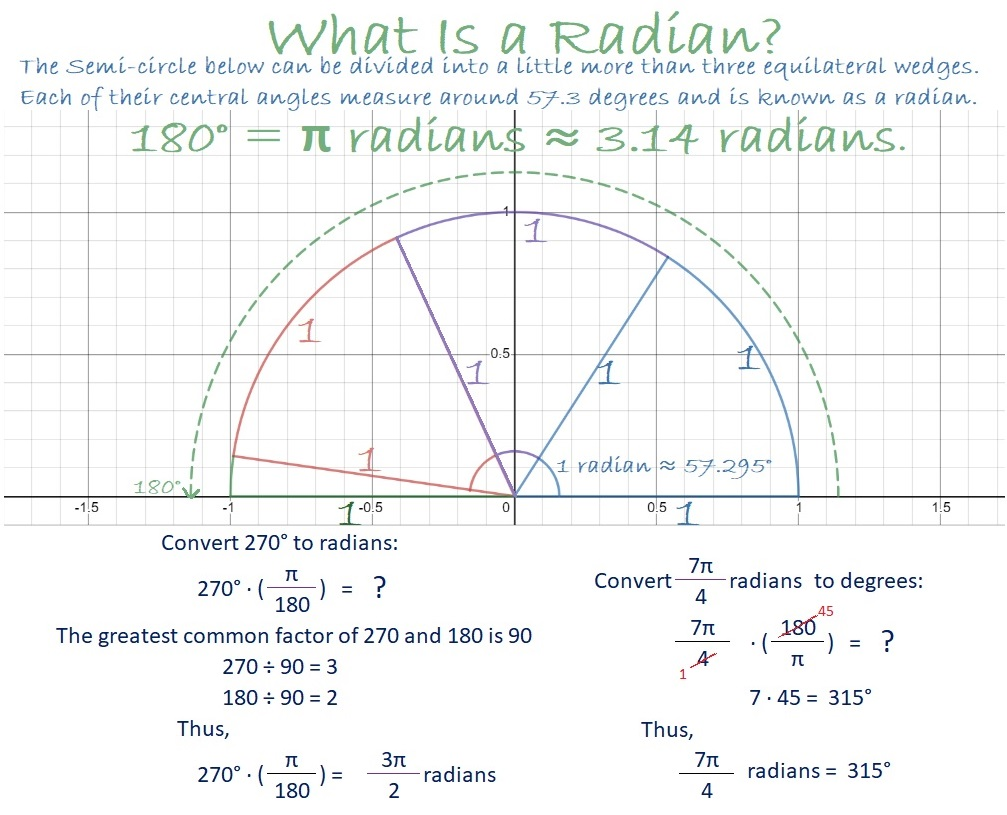Since I have students who rely on calculators but don’t have access to one during this pandemic, I designed a calculator in Desmos to convert Degrees to and from Radians. The calculator doesn’t work like magic, still requires you to think about what you are doing, and should prevent some of the common errors in doing such conversions.

### Factors of 1465:

• 1465 is a composite number.
• Prime factorization: 1465 = 5 × 293
• 1465 has no exponents greater than 1 in its prime factorization, so √1465 cannot be simplified.
• The exponents in the prime factorization are 1, and 1. Adding one to each exponent and multiplying we get (1 + 1)(1 + 1) = 2 × 2 = 4. Therefore 1465 has exactly 4 factors.
• The factors of 1465 are outlined with their factor pair partners in the graphic below.### More Facts about the Number 1465:

1465 is the sum of two squares in two different ways:
36² + 13² = 1465
32² + 21² = 1465

1465 is also the hypotenuse of FOUR Pythagorean triples:
340-1425-1465 which is 5 times 68-285-293.
583-1344-1465 calculated from 36² – 13², 2(36)(13), 36² + 13².
879-1172-1465 which is (3-4-5) times 293.
936-1127-1465 calculated from 2(36)(13), 36² – 13², 36² + 13².

From Stetson.edu, we learn this fun square number fact:
1465² = 2146225.

# 1464 Alibis and a Mystery

### Today’s Puzzle:

You find clues 30, 30, 30, 54. Two of those 30’s claim to be 3 × 10.
When you find clues 40, 40, 56, you realize that at least one of the 40’s must be 4 x 10.

Can you believe the alibis the 30’s have just given you?
Can you put your detective skills together to figure out this mystery?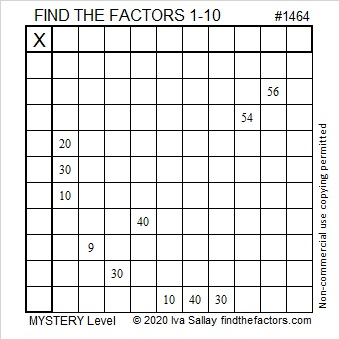### Factors of 1464:

• 1464 is a composite number.
• Prime factorization: 1464 = 2 × 2 × 2 × 3 × 61, which can be written 1464 = 2³ × 3 × 61
• 1464 has at least one exponent greater than 1 in its prime factorization so √1464 can be simplified. Taking the factor pair from the factor pair table below with the largest square number factor, we get √1464 = (√4)(√366) = 2√366
• The exponents in the prime factorization are 3,1 and 1. Adding one to each exponent and multiplying we get (3 + 1)(1 + 1) (1 + 1) = 4 × 2 × 2 = 16. Therefore 1464 has exactly 16 factors.
• The factors of 1464 are outlined with their factor pair partners in the graphic below.### More Facts about the Number 1464:

1464 is the hypotenuse of a Pythagorean triple:
264-1440-1464 which is 24 times (11-60-61).

Stetson.edu alerts us that 1464 is a repdigit in two different bases:
It’s 1111 in BASE 11 because 11³ + 11² + 11¹ + 11º = 1464, and
it’s 888 in BASE 13 because 8(13² + 13¹ + 13º) = 8(183) =1464.

# 1463 When to Use the Law of Sines and the Law of Cosines

For years I have taught my students the law of sines and the law of cosines using only a hand-held scientific calculator. Unfortunately, many of them don’t have access to one during the pandemic. I found some online law of sines and law of cosines calculators, but I felt like they all seemed to find solutions by magic, without requiring any understanding. I want my students to understand when to use the law of sines and when to use the law of cosines.

### When Should I use the Law of Sines?

Use the law of sines when you are given these three pieces of information about a triangle: Angle-Angle-Side, Angle-Side-Angle, or Angle-Side-Side. Here’s a graphic I made showing each case and how the law of sines is transformed to obtain the missing angle or side: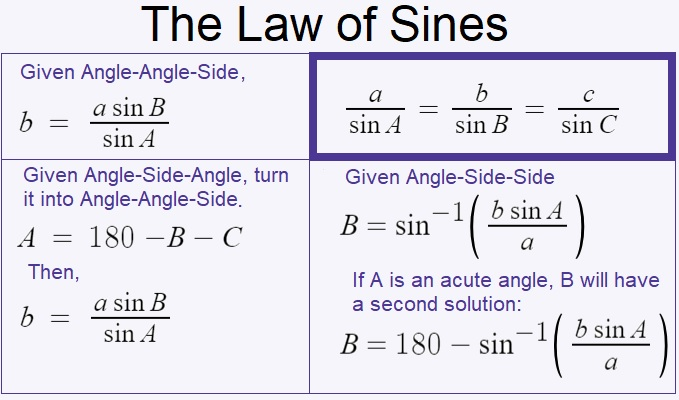I used Desmos to make a Law of Sines calculator that uses the above equations derived from the law of sines. Angles will be entered and given in degrees, not radians. This Law of Sines calculator still forces you to think about what information you’re given and which equation you should use. In your problem, there will be a known angle and a known side that are opposite to each other. Label these known values A and a, respectively. If you have another angle, label it B. If you have another side, label it b. Find the appropriate equation in the calculator, replace the variables with their values, and Desmos will calculate the answer. If you’re given Angle-Side-Side, you will need to use the inverse sine function, and you might have two solutions. Thus, if the given angle is acute, be sure to replace “Bacute” with the acute angle found in the formula above it so that you will also have that second solution. A triangle can’t have two obtuse angles, so don’t give two solutions for B if A is obtuse!

Some people might try to add sliders to that calculator because then they won’t have to think as much. Adding sliders would confuse Desmos with too many variables, so I also made a law of sines calculator with sliders. Angles will be entered and given in degrees, not radians. In your problem, there will be a known angle and a known side that are opposite to each other. Label these known values A and a, respectively. If you have another angle, label it B. If you have another side, label it b. Instead of typing the values into the equation, you can type the values in as sliders. The default for all the sliders is set at 0, so if any of your solutions is 0 or undefined, you are either looking in the wrong place for your answer or you haven’t entered enough information. If you use that calculator, you will need to refresh and reload it after each problem.

### When Should I use the Law of Cosines?

The Law of Cosines is easy enough to type: c² = a² + b² – 2ab cos C. It looks a little like the Pythagorean Theorem, and when C is 90º, that’s exactly what it is.

Use the law of cosines when you are given these three pieces of information about a triangle: Side-Angle-Side or Side-Side-Side. Here’s a graphic I made showing both cases:Since the Law of Cosines requires both a and b to be entered twice, I decided to go ahead and use sliders in the Law of Cosines calculator to make typing a little easier. Angles will be entered and given in degrees, not radians. If you are given Side-Angle-Side, let the angle be C. If you are given Side-Side-Side, label the side opposite the angle you are looking for, c. It doesn’t matter which side you label a or b, but that opposite side must be c. You will need to use the inverse cosine function, but I already have that in the calculator for you. You will need to refresh and reload before starting a new problem.

Since this is post number 1463, I’ll write a little bit about that number.

### Factors of 1463:

Since both 14 and 63 are multiples of 7, we can be sure that 1463 is divisible by 7.

• 1463 is a composite number.
• Prime factorization: 1463 = 7 × 11 × 19
• 1463 has no exponents greater than 1 in its prime factorization, so √1463 cannot be simplified.
• The exponents in the prime factorization are 1, 1, and 1. Adding one to each exponent and multiplying we get (1 + 1)(1 + 1)(1 + 1) = 2 × 2 × 2 = 8. Therefore 1463 has exactly 8 factors.
• The factors of 1463 are outlined with their factor pair partners in the graphic below.Since all four of the factor pairs of 1463 contain only odd numbers, 1463 can be written as the difference of two squares based on those four ways:

732² – 731² = 1463 (732 is the average of 1 and 1463, and 731 is one less.)
108² – 101² = 1463 (108 is the average of 7 and 209, and 101 is seven less.)
72² – 61² = 1463 (72 is the average of 11 and 133, and 61 is eleven less.)
48² – 29² = 1463 (48 is the average of 19 and 77, and 29 is nineteen less.)

# 1462 Has a Palindromic Factor Pair

There are only forty-five numbers between 100 and 10,000 that have palindromic factor pairs. That’s really not very many.

1462 is one of those numbers. That is pretty amazing, all by itself, but 1462 is also only four numbers more than another number on the list. That’s even more amazing!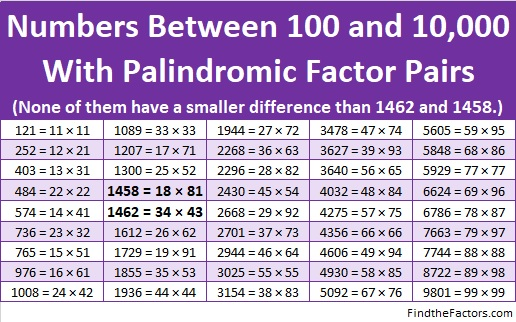Here are some more facts about the number 1462:

• 1462 is a composite number.
• Prime factorization: 1462 = 2 × 17 × 43
• 1462 has no exponents greater than 1 in its prime factorization, so √1462 cannot be simplified.
• The exponents in the prime factorization are 1, 1, and 1. Adding one to each exponent and multiplying we get (1 + 1)(1 + 1)(1 + 1) = 2 × 2 × 2 = 8. Therefore 1462 has exactly 8 factors.
• The factors of 1462 are outlined with their factor pair partners in the graphic below.1462 is the hypotenuse of one Pythagorean triple:
688-1290-1462 which is (8-15-17) times 86

# 1461 and Level 6

### Today’s Puzzle:

Look at all the clues in this level 6 puzzle. Can you deduce the logic needed to begin solving it? Deduce it correctly, then solving the rest of the puzzle will be a breeze.### Factors of 1461:

• 1461 is a composite number.
• Prime factorization: 1461 = 3 × 487
• 1461 has no exponents greater than 1 in its prime factorization, so √1461 cannot be simplified.
• The exponents in the prime factorization are 1, and 1. Adding one to each exponent and multiplying we get (1 + 1)(1 + 1) = 2 × 2 = 4. Therefore 1461 has exactly 4 factors.
• The factors of 1461 are outlined with their factor pair partners in the graphic below.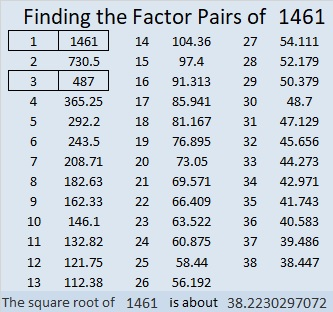1461 is the difference of two squares in two different ways:
731² – 730² = 1461
245² – 242² = 1461

# 1460 and Level 5

### Today’s Puzzle:

Can you use logic to figure out where the numbers from 1 to 10 must go so that the given clues and the factors you find make this puzzle a multiplication table?### Factors of 1460:

• 1460 is a composite number.
• Prime factorization: 1460 = 2 × 2 × 5 × 73, which can be written 1460 = 2² × 5 × 73
• 1460 has at least one exponent greater than 1 in its prime factorization so √1460 can be simplified. Taking the factor pair from the factor pair table below with the largest square number factor, we get √1460 = (√4)(√365) = 2√365
• The exponents in the prime factorization are 2, 1, and 1. Adding one to each exponent and multiplying we get (2 + 1)(1 + 1)(1 + 1) = 3 × 2 × 2 = 12. Therefore 1460 has exactly 12 factors.
• The factors of 1460 are outlined with their factor pair partners in the graphic below.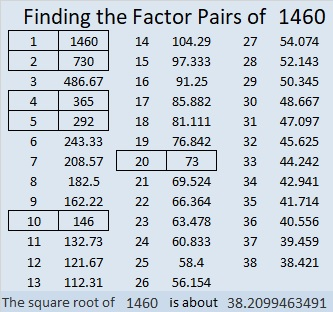As I mentioned earlier, √1460 = 2√365.
Most years have 365 days in them, but not 2020, our current year. Nevertheless,
1460 ÷ 73 × 101= 2020.

1460 is the sum of two squares in two different ways:
28² + 26² = 1460
38² + 4² =1460

1460 is the hypotenuse of FOUR Pythagorean triples:
108-1456-1460, calculated from 28² – 26², 2(28)(26), 28² + 26²
304-1428-1460, calculated from 2(38)(4), 38² – 4², 38² + 4²
876-1168-1460 which is (3-4-5) times 292
960-1100-1460 which is 20 times (48-55-73)